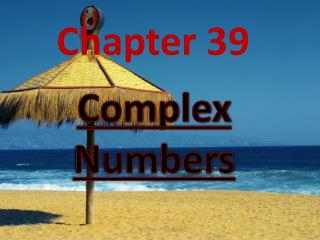DownloadDownload PresentationChapter 39

# Chapter 39

Download Presentation## Chapter 39

- - - - - - - - - - - - - - - - - - - - - - - - - - - E N D - - - - - - - - - - - - - - - - - - - - - - - - - - -
##### Presentation Transcript

1. Chapter 39 Complex Numbers By Chtan FYHS-Kulai

2. By Chtan FYHS-Kulai

3. consist of all positive and negative integers, all rational numbers and irrational numbers. By Chtan FYHS-Kulai

4. Rational numbers are of the form p/q, where p, q are integers. Irrational numbers are By Chtan FYHS-Kulai

5. Complex number notation By Chtan FYHS-Kulai

6. Algebra form Trigonometric form Notation index form By Chtan FYHS-Kulai

7. Complex numbers are defined as numbers of the form : or By Chtan FYHS-Kulai

8. is represented by are real numbers. A complex number consists of 2 parts. Real part and imaginary part. By Chtan FYHS-Kulai

9. Notes: • When b=0, the complex number is Real • When a=0, the complex number is imaginary • The complex number is zero iff a=b=0 By Chtan FYHS-Kulai

10. VENN DIAGRAMRepresentation • All numbers belong to the Complex number field, C. The Real numbers, R, and the imaginary numbers, i, are subsets of C asillustrated below. Complex Numbers a + bi Real Numbers a + 0i Imaginary Numbers 0 + bi

11. Conjugate complex number By Chtan FYHS-Kulai

12. Conjugate complex numbers The complex numbers and are called conjugate numbers. By Chtan FYHS-Kulai

13. is conjugate of . By Chtan FYHS-Kulai

14. e.g. 1 Solve the quadratic equation Soln: By Chtan FYHS-Kulai

15. e.g. 2 Factorise . Soln: By Chtan FYHS-Kulai

16. Representation of complex number in an Argand diagram By Chtan FYHS-Kulai

17. (Im) y P(a,b) x (Re) 0 P’(-a,-b) Argand diagram By Chtan FYHS-Kulai

18. e.g. 3 If P, Q represent the complex numbers 2+i, 4-3i in the Argand diagram, what complex number is represented by the mid-point of PQ? By Chtan FYHS-Kulai

19. y (Im) Soln: P(2,1) x (Re) 0 Q(4,-3) Mid-point of PQ is (3,-1) is the complex number. By Chtan FYHS-Kulai

20. By Chtan FYHS-Kulai

21. Do pg.272 Ex 20a By Chtan FYHS-Kulai

22. Equality of complex numbers By Chtan FYHS-Kulai

23. The complex numbers and are said to be equal if, and only if, a=c and b=d. By Chtan FYHS-Kulai

24. e.g. 4 Find the values of x and y if (x+2y)+i(x-y)=1+4i. Soln: x+2y=1; x-y=4 2y+y=1-4; 3y=-3, y=-1 x-(-1)=4, x=4-1=3 By Chtan FYHS-Kulai

25. Addition of complex numbers By Chtan FYHS-Kulai

26. If then By Chtan FYHS-Kulai

27. Subtraction of complex numbers By Chtan FYHS-Kulai

28. If then By Chtan FYHS-Kulai

29. Do pg.274 Ex 20b By Chtan FYHS-Kulai

30. Multiplication of complex numbers By Chtan FYHS-Kulai

31. e.g. 5 If , find the values of (i) (ii) Soln: (i) (ii) By Chtan FYHS-Kulai

32. If then By Chtan FYHS-Kulai

33. Division of complex numbers By Chtan FYHS-Kulai

34. If then By Chtan FYHS-Kulai

35. e.g. 6 Express in the form . Soln: By Chtan FYHS-Kulai

36. e.g. 7 By Chtan FYHS-Kulai

37. e.g. 8 If z=1+2i is a solution of the equation where a, b are real, find the values of a and b and verify that z=1-2i is also a solution of the equation. By Chtan FYHS-Kulai

38. The cube roots of unity By Chtan FYHS-Kulai

39. If is a cube root of 1, By Chtan FYHS-Kulai

40. By Chtan FYHS-Kulai

41. Notice that the complex roots have the property that one is the square of the other, By Chtan FYHS-Kulai

42. let So the cube roots of unity can be expressed as By Chtan FYHS-Kulai

43. If we take then or vice versa. By Chtan FYHS-Kulai

44. (1) As is a solution of (2) As is a solution of By Chtan FYHS-Kulai

45. (3) (4) (5) … etc By Chtan FYHS-Kulai

46. e.g. 9 Solve the equation . By Chtan FYHS-Kulai

47. e.g. 10 By Chtan FYHS-Kulai

48. Soln: By Chtan FYHS-Kulai

49. Do pg.277 Ex 20c By Chtan FYHS-Kulai

50. The (r,θ) form of a complex number By Chtan FYHS-Kulai